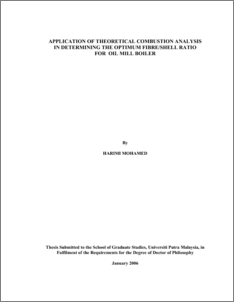# Application of Theoretical Combustion Analysis in Determining the Optimum Fibre/Shell Ratio for Oil Mill Boiler

## Citation

Mohamed, Harimi (2006) Application of Theoretical Combustion Analysis in Determining the Optimum Fibre/Shell Ratio for Oil Mill Boiler. PhD thesis, Universiti Putra Malaysia.

## Abstract

Currently from 50 million tons of fibre and shell generated yearly, only 60% are used in all palm oil mills as solid fuel for steam boilers. The only problem when incinerating the fibre and shell in a random mixture, the amount of air required would be difficult to control or to maintain and consequently, the control of flue gas emissions become the most difficult task. If the excess air is well optimised with respect to a given ratio of fibre-to-shell, then whatever the variation of that ratio, the appropriate amount of air should be allotted to that mixing [fibre and shell] based on the optimisation value of excess air. In this study, five different compositions of fibre and shell were obtained from five different palm oil mills in Malaysia, and have been tested with other data obtained from Malaysian Palm Oil Board [MPOB] database. The results confirmed statistically, that those data obtained from the five different palm oil mills represent the Malaysian local data.Twenty-six chemical species were selected, and based on chemical reaction stoichiometry method [CRS] and chemical equilibrium reactions [CER], the stability of the system was determined. It was found that, the 26 chemical species should be listed from major species to minor species when using CRS in order to get all CER independent from each other, and thus system is more stable. With the existence of powerful software STANJAN code, the emissions of flue gases were computed using all the data from the five palm oil mills. Huge data output were obtained, and therefore minimisation of data was carried out using statistical method namely analysis of variance [ANOVA], and from which the difference between the data output from the five palm oil mills are statistically not significant, and therefore palm oil mill number three was selected as base data. The thermal analysis of heat losses from boiler was presented in details based on direct and indirect method. The second method was used based on ASME PTC4.1 standard, where the first five heat losses were considered, because fibre and shell contain low ash content and therefore heat loss from this source is not so critical. For the heat loss due to radiation and convection, maximum of 2% of heat loss are counted. The main reason of not considering the last two heat losses is also because, many practical data are needed and specially the heat loss due to ash which is really another topic of research. The results obtained from STANJAN code were validated, using theory of combustion based on major chemical species, and also with some practical data from palm oil mills in Malaysia. The output from STANJAN code is of great importance either for theory or practical side. The validation of the results obtained using ASME PTC4.1 standard were valid with the validity of the results obtained from STANJAN software, and also related to the properties of the air. The properties of the air were taken as an average value of temperature and humidity of 28 oC and 80% respectively; therefore the results obtained are acceptable within that average. For the part of boiler thermal analysis, it was observed that the heat loss due dry flue gases is the most dominant heat loss, except at very high fibre content of 60% and above, where the heat loss due to moisture in the fuel takes place. The heat loss due to incomplete combustion was found highly affected by the variation in excess air, but not too much affected by the ratio of fibre-to-shell. The t-test confirmed that the heat loss due to moisture and hydrogen in the fuel was found much more affected by the variations of fibre-to-shell ratios. The mathematical models of CO, CO2, combustion efficiency, and boiler efficiency with respect to percentage excess air and ratio of fibre-to shell were obtained. From the mathematical model of CO, the relation of excess air with respect to the ratio of fibre-toshell was deduced mathematical and numerically by fixing the concentration of CO to 9 ppmv [DOE]. From the data obtained from the mathematical models of the optimum value of excess air with respect to the ratio of fibre-to-shell, it was found that the combustion efficiency is constant at any excess air, and thus the optimum point of fibreto- shell ratio of 55:45 was computed based on the average value of the boiler efficiency,with 75% excess air. The palm oil owners or users are not forced to use such optimum point, but for any given ratio of fibre-to-shell, its appropriate percentage of excess air should be maintained, so that high combustion efficiency and less heat losses are attained.Preview
PDF
548992_FK_2006_30.pdfView Item